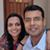D

#### Page 21: If a woman has a mass of 50 kg, calculate her weight in newtons.

62 viewed last edited 3 years agoPrince Walker
1
Let me explain, the acceleration of gravity is g, the weight is F sub g ,and the mass is m. g being an acceleration has to be in between two forces right? But I only see one other one. Also the acceleration and weight are vectors moving downward. Them going in the same direction means that the g is speeding up. Okay, just an observation. Anyways g is acceleration due to gravity, but what is accelerating? I would really prefer this being answered by a Qalaxia person because they are adults, them or Craig.
What I am having difficulty is with the diagram, or step 1 of the 12 step problem solving process.Craig Young
3

You are confusing the vectors involved in speeding up and slowing down. Objects speed up when their VELOCITY and acceleration vectors point the same way, NOT force and acceleration vectors. The gravity vector and the acceleration due to gravity always both point down (toward the center of the Earth).

Your statement that acceleration has to be between two forces is incorrect. FORCES are interactions between two OBJECTS. Remembering back to our kinematics questions, an object by itself can speed up, slow down or turn, so an object by itself can accelerate. The force causing that acceleration (for falling objects) is the gravitational interaction between the Earth and the second object.2

By Newton's second law, F=\ m.a. A force F, acting on a mass m produces an acceleration a.

Weight is a force, caused by gravitation.

By Newton's law of gravitation (smart chap, this Newton), we know that the gravitational force F_g is proportional to the product of the masses of the two bodiesm1.m2 (here, Earth and the person).

F_g\ =\ k.\ m1.m2

Thus, we can see that Earth's gravity causes a constant acceleration (think of both equations together, in case it isn't obvious).

Now, given that the acceleration is g, what is the force that caused it? Multiply it by the mass of the object subject to the acceleration, of course.

Thus, the woman weighs

50\ Kg\ *\ 9.8\ m/s^2=490\ N

acceleration has to be in between two forces right?

No.

Also the acceleration and weight are vectors moving downward. Them going in the same direction means that the g is speeding up.

Yes and No. Acceleration is proportional to Force (weight), so they are in the same direction by definition. g cannot speedup, since it's an acceleration. However, an object under g (that's all of us!) will speed up. Why are we all not speeding up then? That's because we've got a hard surface (the ground) pushing back at us with the same force, balancing out the gravitational force.Mahesh Godavarti
1

Prince, there are many errors in your understanding.

1. "g being an acceleration has to be in between two forces”. Are you thinking for an object to accelerate there have to be two forces and one of them has to be more than the other for the object to accelerate?
2. “g” being acceleration due to gravity might be what is confusing you. Think of this as the acceleration you would experience if nobody was holding you in place. The Earth is always applying a force on you pulling you towards itself and either the ground or the floor is holding you up, keeping you in place. If there were no ground or no floor (i.e, you are in free fall) then you would accelerate with a rate that is equal to g. When you are not accelerating anywhere then the total force being applied on you is 0N. The force due to gravity is still equal to “m g”. That does not change. Except that there is another normal force acting in the opposite direction opposing your weight and holding you in place.
3. "Weight and g being in the same direction mean that the g is speeding up”. The acceleration “g” is caused by the weight therefore, it has to be in the same direction.

Let’s take another example.

Let’s say there is an object of mass m on a very smooth floor. Let’s say you apply a force that will cause the object to accelerate with acceleration a. Then the amount of force you are applying is "m a".

Now, consider the case where your friend is applying an equal and opposite force keeping the object motionless. How much force are you applying? You are still applying the same force as earlier except that the object is no longer accelerating. The force you are applying is still “m a” even though there is no acceleration.

Essentially, acceleration due to gravity is the acceleration you will experience when you are in free fall (when nothing is holding you in place preventing you from falling).

I hope that clears things up.Cesar Centeno
0Mahesh Godavarti
0
Cesar, are you trying to submit your homework?